# Electronics and Communication Engineering - Exam Questions Papers

1.

8416 - 2A16 is equal to

 A. 5A16 B. 4A16 C. 7A16 D. 15A16

Explanation:

(2A)16 = (00101010)2

2's complement = 11010110 = (D6)16

(84)16 + (D6)16 ⇒ 1(5A)16 carry discarded ⇒ (5A)16 .

2.

Calculate the electrostatic force of repulsion between two mica particles at a distance of 10-13 m from each other. Charge on a particle is 3.2 x 10-19 C. (relative permittivity for mica is 5)

 A. 1.843 x 10-15 N B. 9.2 x 10-2 N C. 14.41 x 10-9 N D. none of these

Explanation:

By Coulomb's law:

Electrostatic force =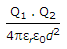=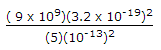= 18.43 x 10- 3 N.

3.

An electromechanical closed loop control system has the following characteristics equation :s3 + 6Ks2 + (K + 2)s = 8
where K is the forward gain of the system. The condition for closed loop stability is

 A. K = 0.528 B. K = 2 C. K = 0 D. K = -2528

Explanation:

s3 + 6ks2 + (k + 2)s + 8 = 0

a0 = 1, a1 = 6k, a2 = k + 2, a3 = 8

Apply R.H. criteria.

4.

What is the propagation constant for air filled wave guide with dimensions a = 1.59" and b = 0.795" at 4.95 GHz. The wave impedance is

 A. 400.94 Ω B. 562.94 Ω C. 502.80 Ω D. 492.80 Ω

Explanation: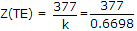.

5.

An antenna array comprises of two dipoles that are separated by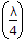wavelength. The dipoles are fed by currents of the same magnitude and 90° in phase. The array factor is:

 A. 2 cos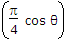- 2 sinB. 2 cos+ 2 sinC. 2 cosD. 2 sinExplanation:

Array factor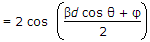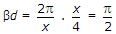φ = 90°.